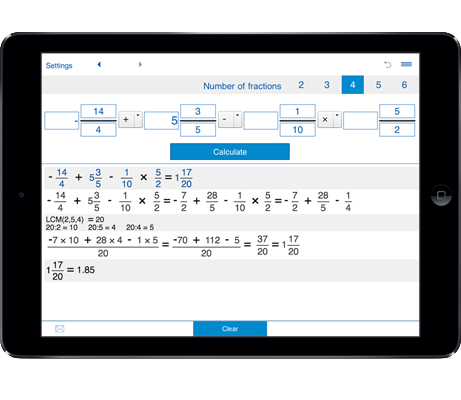# Calculator for fractions

You can use fraction calculator to add, subtract, multiply, and divide two, three, four, five, and six fractions, including proper and improper fractions, integers and mixed numbers.
Shows the details of calculation.
History tape to view your recent calculations.

## Calculator for operations with fractions

Calculator for operations with 2 fractions, 3 fractions, 4 fractions, 5 fractions, and 6 fractions: adding, subtracting, multiplying, and dividing.

### How to use the calculator for fractions

1. Select the number of fractions - 2 fractions, 3 fractions, 4 fractions, 5 fractions, or 6 fractions.

2. Enter the proper or improper fractions, integers and mixed numbers in the provided fields.

For negative fractions put a minus sign in front of a fraction.

3. Select the operations: + adding, - subtracting, * multiplying, / dividing

4. Click the "Calculate" button.